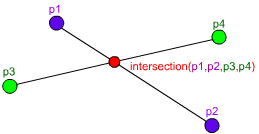## Archive for the ‘C++’ Category

### Line-Line Intersection in C++

Monday, November 3rd, 2008I’ve just been tasked with creating a function to get the intersection of two lines.
With the help of equations from Wolfram Mathworld I created this nifty function:

```Point* intersection(Point p1, Point p2, Point p3, Point p4) {
// Store the values for fast access and easy
// equations-to-code conversion
float x1 = p1.x, x2 = p2.x, x3 = p3.x, x4 = p4.x;
float y1 = p1.y, y2 = p2.y, y3 = p3.y, y4 = p4.y;

float d = (x1 - x2) * (y3 - y4) - (y1 - y2) * (x3 - x4);
// If d is zero, there is no intersection
if (d == 0) return NULL;

// Get the x and y
float pre = (x1*y2 - y1*x2), post = (x3*y4 - y3*x4);
float x = ( pre * (x3 - x4) - (x1 - x2) * post ) / d;
float y = ( pre * (y3 - y4) - (y1 - y2) * post ) / d;

// Check if the x and y coordinates are within both lines
if ( x < min(x1, x2) || x > max(x1, x2) ||
x < min(x3, x4) || x > max(x3, x4) ) return NULL;
if ( y < min(y1, y2) || y > max(y1, y2) ||
y < min(y3, y4) || y > max(y3, y4) ) return NULL;

// Return the point of intersection
Point* ret = new Point();
ret->x = x;
ret->y = y;
return ret;
}```

Hope it will be to as good use to you as it is to me.